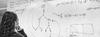# 2nd Semester 2006/07: Translating Between Logics

Instructors
Theo Janssen
ECTS
6
Description
There are several translations between logics, e.g. between modal logic and predicate logic, and between classical logic and intuitionistic logic. A surprising example of a translation is given by Béziau (1999). He considers PL/2 which is propositional logic (PL) with only one half of the meaning of negation, and provides a translation from PL into the seemingly weaker logic PL.

One might ask when a translation between logics is a correct translation, and under which circumstances such a translation is possible at all. A general perspective on this issue is given in Carnielli & d'Ottaviano (1997) and Feitosa & d'Ottaviano (2001). A reaction on this is Janssen (2007). The proposals in these papers are of algebraic nature. One might expect that a correct translation from a logic that makes fine distinctions into a logic that makes few distinctions is not possible (because in the translation essential information is lost). But it is reasonable to expect that a translation from a logic that makes few distinctions (propositional logic) into a logic with finer distinctions (intuitionistic propositional logic) is possible (the G�del translation).

Problem. (Humberstone 2005) gives a counterexample to the intuitive result mentioned above. One of the involved logics is KT, which is the basic normal logic K extended with BoxA implies A. The other is the minimal modal logic L. He shows that there is no translation (satisfying certain requirements) from KL into L, whereas on intuitive considerations one would expect this to be easy.